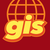# Create ServiceLines automatically?

225
3
03-30-2022 07:12 AMNew Contributor II

Hi everybody,

I just wonder if there is any way or any tool which helps me to create ServiceLines (Laterals) automatically within my UtilityNetwork. As fas as I remember, there was such a Tool in the previous Geometric Network fo ArcMap.

Some ideas about how to do it?

Tags (3)
3 RepliesbyEsri Frequent Contributor

We have played around doing this in Arcade and an attribute rule.

Here is the code if you want to try it out.

``````function find_closest_line() {
var line_class = FeatureSetByName(\$datastore, "line", ["objectid"], true);
//var candidates = Intersects(line_class, Buffer(\$feature, 500, "miles"));
var candidates = line_class;

var shortest = [1e10, null];
for (var line in candidates) {
var d = Distance(\$feature, line)
if (d < shortest) shortest = [d, line]
}

return shortest[-1]
}

function point_and_distance(point_feature, other_feature) {
/*
finds the closest point from point_feature to other_feature

Args:
point_feature: Point Geometry
other_feature: Point/Line/Polygon Geometry

Returns: dictionary
{distance: number,               // distance from point_feature to closest point
point: geometry,  // the coordinate pair of the closest point
isVertex: bool}                 // if the closest point is a vertex of other_feature

*/

var point_feature = Geometry(point_feature);
var other_feature = Geometry(other_feature);

var shape = TypeOf(other_feature);
var vertices;
if (shape == 'Point') {
return {"distance": Distance(point_feature, other_feature),
"coordinates": [other_feature["x"], other_feature["y"]],
"isVertex": true}
}

else if (shape == 'Multipoint') {
var points = other_feature["points"];
var shortest = [1e10, null];
for (var i in points) {
var p = points[i]
var dist = Distance(point_feature, p);
if (dist < shortest) shortest = [dist, [p["x"], p["y"]]]
}
return {"distance": shortest,
"coordinates": shortest,
"isVertex": true}
}

else if (shape == 'Polyline') vertices = other_feature["paths"]
else if (shape == 'Polygon') vertices = other_feature["rings"]
else return null

var x = point_feature["x"];
var y = point_feature["y"];

// Loop through each part of the geometry and each segment, tracking the shortest distance
var shortest = [1e10];
for (var i in vertices) {
var part = vertices[i];
var previous = part;
for (var j = 1; j < Count(part); j++) {
var current = part[j];
var result = pDistance(x, y, previous["x"], previous["y"], current["x"], current["y"]);
if (result < shortest) shortest = result
previous = current;
}

}

// Couldn't find anything
if (Count(shortest) == 1) return null

return {"distance": shortest,
"coordinates": shortest,
"isVertex": shortest}

}

function pDistance(x, y, x1, y1, x2, y2) {
var A = x - x1;
var B = y - y1;
var C = x2 - x1;
var D = y2 - y1;

var dot = A * C + B * D;
var len_sq = C * C + D * D;
var param = -1;
if (len_sq != 0) //in case of 0 length line
param = dot / len_sq;

var xx, yy;
var is_vertex = true;
if (param < 0) {
xx = x1;
yy = y1;
}
else if (param > 1) {
xx = x2;
yy = y2;
}
else {
is_vertex = false;
xx = x1 + param * C;
yy = y1 + param * D;
}

var dx = x - xx;
var dy = y - yy;
return [Sqrt(dx * dx + dy * dy), [xx, yy], is_vertex];
}

function create_line(start, end_coordinates) {
var geo = Geometry(start);
return Polyline({"paths": [[[geo["x"], geo["y"]], end_coordinates]], "spatialReference": geo["spatialReference"]})

}

var closest = find_closest_line();
if (closest == null) return null

var data = point_and_distance(\$feature, closest);
if (data == null) return null

var lateral = create_line(\$feature, data["coordinates"]);

return {"result": null,
"edit": [{
}
]
}``````New Contributor II

Thanks for the answer Mike, I would like to have a more firendly user tool within the UN (as in the previous Geometric Network) but I will have to try it this way if no other option then.
Thanks for the code and I will write a feedback if I finally have to use itOccasional Contributor II

I'll be trying that, I also really like those ArcMap tools Sergio mentions, thanks Mike.